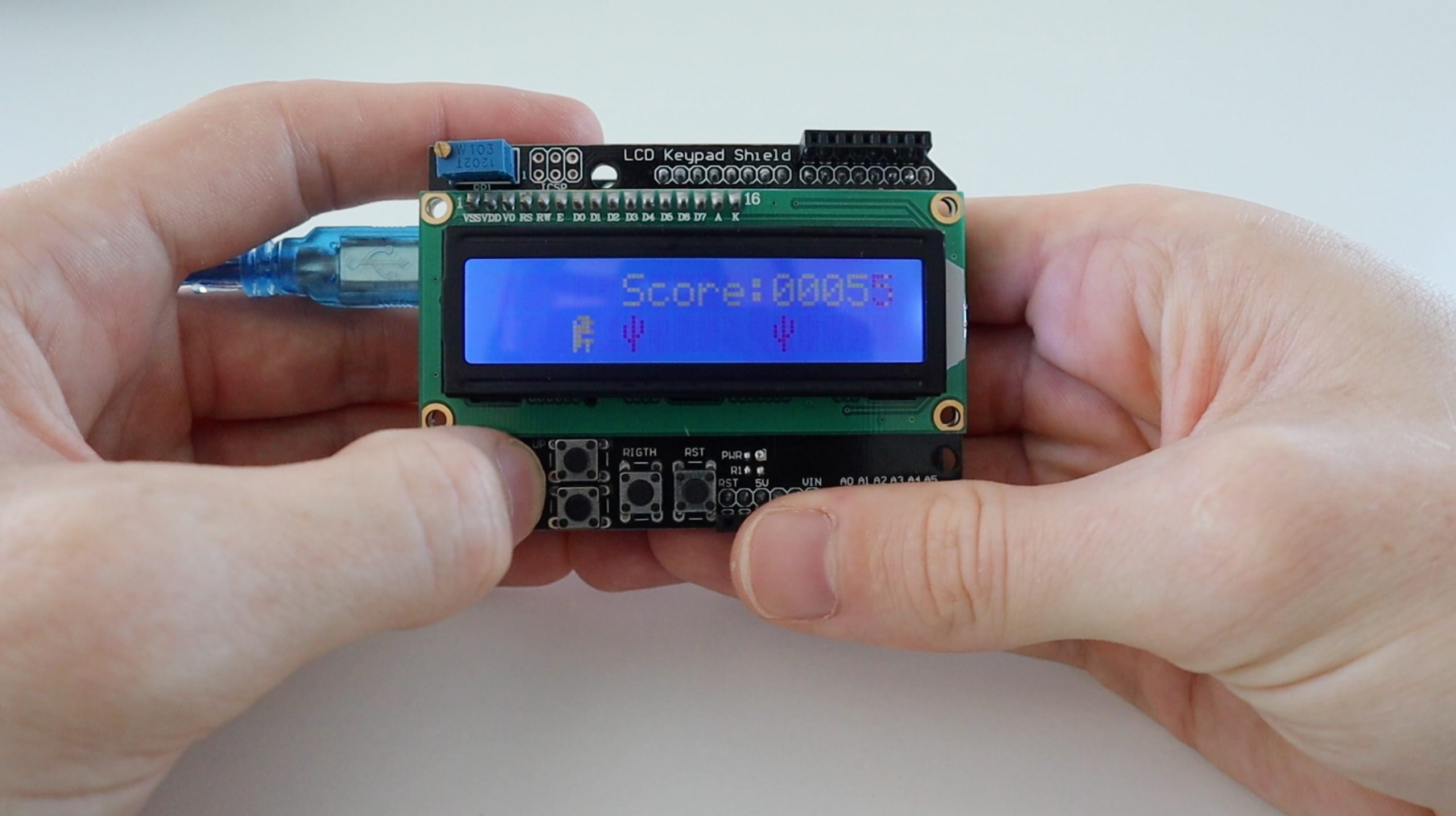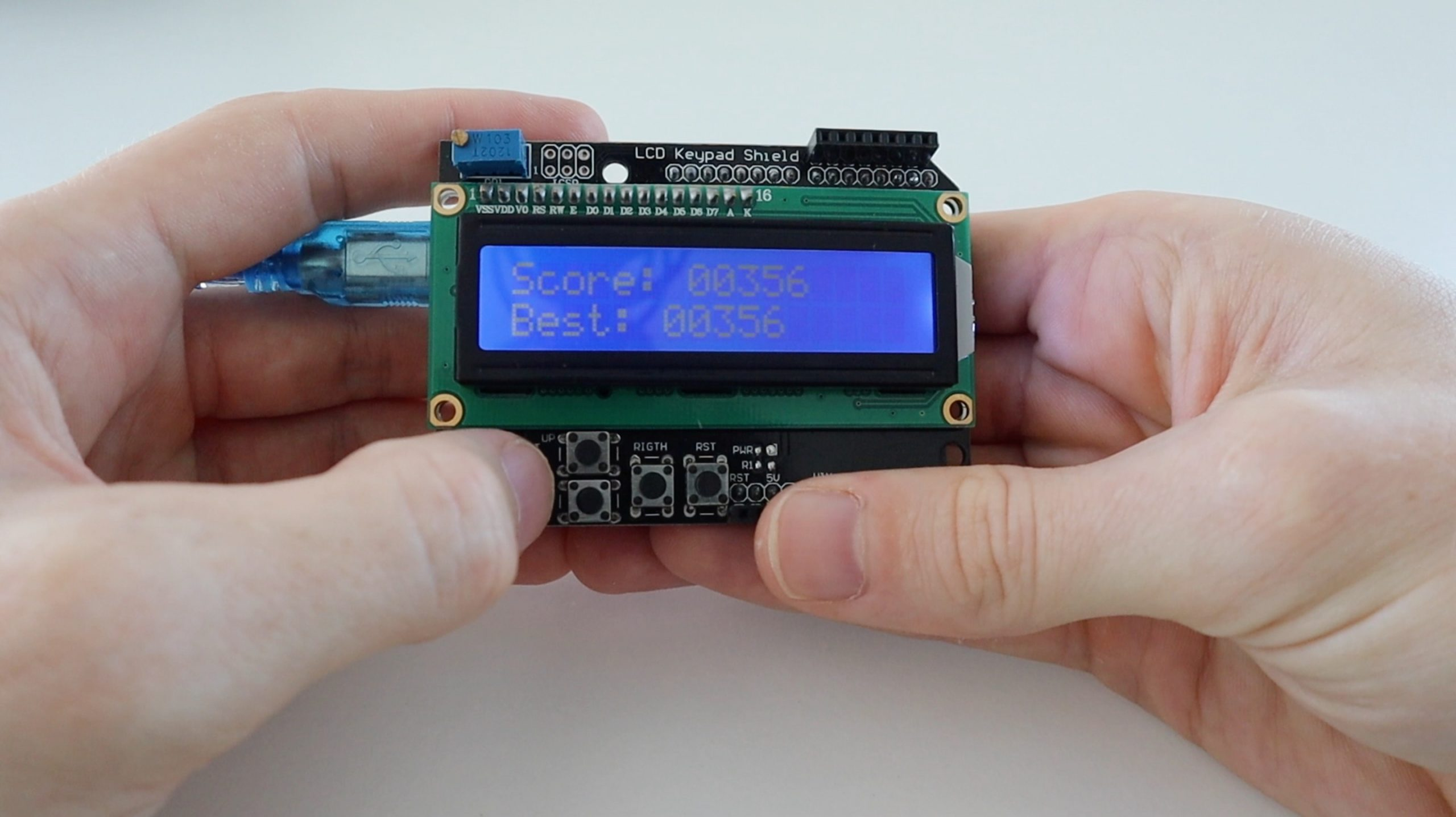If you’ve been kept busy playing the Chrome Dino Game in your browser, how about loading it onto an Arduino to leave on your desk? It’s a really simple game which runs quite well on an Arduino Uno using a simple LCD keypad shield without any other hardware.

I didn’t code this game, it’s a version I found on hackster.io which seems to run the best. It’s written in AVR C code, so it looks a bit different to the generic Arduino language but you should be able to figure a lot of it out and you can still open, edit and upload it using the Arduino IDE.

Here’s a video of the Chrome Dino Game being played on an Arduino:

## What You Need To Run The Chrome Dino Game

First, start off by plugging your LCD keypad shield onto your Arduino Uno. Make sure that all of the pins are lined up correctly so that you don’t bend any of them.

Next, plug the Arduino into your computer and start up the Arduino IDE. Make sure that the correct board is selected and that you’re working on the correct com port. Then upload the below sketch. You can also change a couple of things in the sketch if you like, I’ve outlined these after the sketch.

Here’s a brief overview of the game:

• The game speeds up the longer you play it.
• Cactuses are initially separated by a minimum of 5 spaces and this goes down to a minimum of 3 as the game progresses.
• Cheating by holding down the button or continuously pressing the button is prevented.
• The current score is displayed during the game and the high score at the end.
• The high score is not saved and is lost when power to the Arduino is removed.

Let’s have a look at the code:

```//From Hackster.io
//By BRZI

#include <avr/io.h>
#define  F_CPU 16000000UL //Our CPU speed (16MHz)
#include <util/delay.h> //Libraries for delay and interrupt utilities
#include <avr/interrupt.h>
#define command 0 //explained in dispSend() function
#define write 1

uint8_t upperBuff , downerBuff, overMsgUpper[] = "Score:  ", overMsgDowner[] = "Best:   ", scoremsg[] = "Score:" , din[] = {0x0E, 0x17, 0x1E, 0x1F, 0x18, 0x1F, 0x1A, 0x12}, cact[] = {0x04, 0x05, 0x15, 0x15, 0x16, 0x0C, 0x04, 0x04};
//Buffers for line one and two. Message to display after lost game.                     //Score text during game. //Dinosaur and cactus bitmaps
uint8_t canup = 1, longhold = 0, distance = 6, speed = 200, isup = 0, dontprint = 0; //All of these are explained further
uint16_t aVal = 0, score = 1, bestscore = 0;
int i;

void dispInit();
void dispWrite(uint8_t bits);
void dispSend(uint8_t bits, uint8_t act);
void dispSetLine(uint8_t line);
void dispClear();
void dispHome();
void dispPrintChar(uint8_t chr[], uint8_t size);

int main(void)
{
for(i = 0; i < 17; i++) downerBuff[i] = ' '; //Initialize upper and downward buffer
for(i = 0; i < 17; i++) upperBuff[i] = ' ';

dispInit(); //Initialize the display

TCCR1B |= (1 << WGM12) | (1 << CS11); //Set Timer1 to compare to OCR1A and prescaler of 8
OCR1AH = (500 >> 8); //This equals to 2000Hz or 500us timing, look for TIMER1_COMPA_vect down below
OCR1AL = 500;
TIMSK1 |= (1 << OCIE1A); //Enable Timer1 CompA ISR
sei(); //Enable global interrupt

ADMUX = (1 << REFS0); //Set AREF to VCC

while (1) {

srand(aRead()); //Use it as a random seed
ADMUX &= ~(1 << MUX2) & ~(1 << MUX0); //Revert back to ADC0 to read the button value

if(aRead() > 900) longhold = 0; //Reads if Up button has been released to prevent cheating. The value is so low because if you hold your fingers beneath one of the buttons the voltage would drop, this prevents the dinosaur from locking up

for(i = 0; i < 16; i++) downerBuff[i] = downerBuff[i + 1]; //Shifts everything in downward buffer by one place to the left
if((rand() % 100) > (rand() % 100) && !dontprint){ //This portion decides if it should put a cactus or a blank spot, dontprint is used to prevent cactus grouping
downerBuff = 0x01; //0x01 represents the cactus (we added cactus and dinosaur to CGRAM when we initialized the display)
dontprint = 1; //This part acts both as a boolean and a counter to ensure cactus separation
}
else downerBuff = ' ';
char lastchar = downerBuff; //We remember the whats initially added to the downward buffer before replacing it with the dinosaur
if(!isup){ //If din should be placed down
downerBuff = 0x00; //Place it down
dispSetLine(2);
dispPrintChar(downerBuff, sizeof(downerBuff)); //Draw it
downerBuff = lastchar; //Place back previous thing to the buffer
canup = 1;  //This flag is used to disable dinosaur from getting up before it was drawn down, in this case he can go up
} else { //If din should be placed up
upperBuff = 0x00; //Place it up in upper buff
dispSetLine(1);
dispPrintChar(upperBuff, sizeof(upperBuff));
dispSetLine(2);
dispPrintChar(downerBuff, sizeof(downerBuff)); //Draw it
canup = 0; //In this case he wont go up until rendered on line 2
}

if(dontprint) dontprint++;
if(dontprint > distance) dontprint = 0; //This is the part that ensures cactus separation, it will keep the cactus 3-5 spaces apart minimally (depends on the game progress)

if(isup) isup++; //This part makes sure din is on upper side for 3 loops after he was initially drawn there
if(isup > 4){
upperBuff = ' ';
dispSetLine(1);
dispPrintChar(upperBuff, sizeof(upperBuff));
isup = 0;
}
for(i = 0; i < sizeof(scoremsg); i++) upperBuff[i + 5] = scoremsg[i]; //This part prints the current score during the game
uint8_t cnt = 11;
for(i = 10000; i > 0; i /= 10){
upperBuff[cnt] = ((score / i) % 10) + '0';
cnt++;
dispSetLine(1);
dispPrintChar(upperBuff, sizeof(upperBuff));
}

score++; //Increment the score once on loop
if(score > bestscore) bestscore = score; //Remember best score

if(lastchar == 0x01 && !isup){ //Check if the dinosaur is downward and hit a cactus
dispClear(); //Clear the display and buffers
for(i = 0; i < 17; i++) downerBuff[i] = ' ';
for(i = 0; i < 17; i++) upperBuff[i] = ' ';
uint8_t cnt;

dispSetLine(1);
for(i = 0; i < sizeof(overMsgUpper); i++) upperBuff[i] = overMsgUpper[i]; //Display worst and best score
cnt = sizeof(overMsgUpper) - 1;
for(i = 10000; i > 0; i /= 10){
upperBuff[cnt] = ((score / i) % 10) + '0';
cnt++;
}
dispPrintChar(upperBuff, sizeof(upperBuff));

dispSetLine(2);
for(i = 0; i < sizeof(overMsgDowner); i++) downerBuff[i] = overMsgDowner[i];
cnt = sizeof(overMsgDowner) - 1;
for(i = 10000; i > 0; i /= 10){
downerBuff[cnt] = ((bestscore / i) % 10) + '0';
cnt++;
}
dispPrintChar(downerBuff, sizeof(downerBuff));

while(1){ //Wait for select button to be pressed
if(aVal > 635 && aVal < 645){ //After that clear all the variables
for(i = 0; i < 17; i++) downerBuff[i] = ' ';
dispSetLine(1);
dispPrintChar(downerBuff, sizeof(downerBuff));
for(i = 0; i < 17; i++) upperBuff[i] = ' ';
dispSetLine(2);
dispPrintChar(upperBuff, sizeof(upperBuff));
dontprint = 0;
isup = 0;
score = 1;
speed = 200;
longhold = 0;
distance = 6;
canup = 1;
break;
}
}

}
if(score % 5 == 0) speed -=2; //If score is divisible by 5 make game faster by -2ms
if(speed < 120) speed = 120; //Minimal time in ms (+ ~2ms) that the loop will be halted for (limited by display refreshing, in my testing 11.8Hz was readable enough to be playable)
if(score % 175 == 0) distance--; //Every time you score a number divisible by 175 minimal cactus distance gets smaller
if(distance < 3) distance = 3;
for(i = 0; i < speed; i++) _delay_ms(1); //This is the only way as the compiler expects a const number here
}
}

void dispInit(){
_delay_ms(50); //Just in case
DDRD = 0b11110000; //Set these pins to output. PD4 - PD7 correspond to D4 - D7 on display, we need to configure it to run in 4 bit mode
DDRB = 0b00000011; //PB0 is tied to RS and PB1 to EN
dispWrite(0x30);//*This part here is explained in Hitachi HD44780 datasheet on how to initialize the display in 4bit mode
_delay_us(4500);//*Essentially you send the reset signal 3 times, and then set it to 4 bit mode
dispWrite(0x30);//*
_delay_us(4500);//*
dispWrite(0x30);//*
_delay_us(4500);//*
dispWrite(0x28);//*
dispSend(0x28, command); //Send 4bit mode function set
dispSend(0x08, command); //Turn the display off
dispSend(0x01, command); //Clear its RAM (if MCU resets that doesn't mean the display was reset, so we clear everything)
_delay_ms(50);
dispSend(0x0C, command); //Turn the display on
_delay_ms(5);
dispSend(0x40, command); //Tell the display we want to enter a custom character to its CGRAM (on address 0x00)
for(i=0; i<8; i++) dispSend(din[i], write);
dispSend(0x80, command); //Transaction end
dispSend(0x48, command); //Same thing, but for 0x01
for(i=0; i<8; i++) dispSend(cact[i], write);
dispSend(0x80, command);
}

void dispPrintChar(uint8_t chr[], uint8_t size){
for(uint8_t i = 0; i < size; i++) dispSend(chr[i], write); //Self explanatory
}

void dispSetLine(uint8_t line){
if(line == 2) dispSend(0xC0, command); //Sets the line where 0xC0 is line 2 and 0x80 is line 1
else dispSend(0x80, command);
}

void dispClear(){
dispSend(0x01, command); //Self explanatory
_delay_ms(2); //This command takes longer for the IC to process, this delay is necessary
}

void dispHome(){ //This function isn't used in this application but its there for expandability, it places the cursor on the line 1 column 1
dispSend(0x02, command); //Self explanatory
_delay_ms(2);
}

void dispSend(uint8_t bits, uint8_t act){
if(act) PORTB |= (1 << DDB0); //Set PB0 if we are writing a character, else pull it low
else PORTB &= ~(1<<DDB0);
dispWrite(bits); //Send the bit then shift them 4 bit to the left to work in displays 4bit mode
dispWrite(bits << 4);
_delay_us(80);
}

void dispWrite(uint8_t bits){
PORTD = bits; //This is a dirty way to write it but it's perfect for this application as it's not bulky and PORTD isn't used for anything else anyway
PORTB |= (1<<DDB1); //Pulse the PB1 to signal the IC to read the data
_delay_us(1);
PORTB &= ~(1<<DDB1);
_delay_us(1);
}

}

ISR (TIMER1_COMPA_vect){ //Timer ISR we set up earlier
if(!longhold){ //Return if the Up button was still held
if(aVal > 450 && aVal < 600 && canup){ //Check if Up is pressed and that din was rendered down
isup = 1;
longhold++;
}
}
}```## Things You Can Change In The Game

There are a couple of things you can edit to customise the game without knowing AVC C code, here are some of them:

• Line 11 – Change the score/high score screen text.
• Line 140 – Change by how much the speed increases every score increment of 5, or change the increment.
• Line 141 – Change the maximum speed limit. The original coder suggests nothing less than 120ms a cycle or it becomes unplayable on the LCD.
• Line 142 – Change how often the cactus spacing gets closer together.
• Line 143 – Change the minimum cactus distance.

The game is quite easy to play on the LCD keypad shield although the buttons are not the best for quick presses and the LCD is quite slow, so it starts suffering from ghosting and brightness issues once the cactuses start moving quickly.

Let me know if you’ve loaded this game onto your Arduino Uno and what you’ve changed on it. Enjoy playing it!